• 哈希表c++
千次阅读
2018-09-16 11:43:54
1. 定义

百度百科显示哈希表的定义是：

散列表（Hash table，也叫哈希表），是根据关键码值(Key value)而直接进行访问的数据结构。也就是说，它通过把关键码值映射到表中一个位置来访问记录，以加快查找的速度。这个映射函数叫做散列函数，存放记录的数组叫做散列表

那么将关键码值映射到表中的一个位置，这种方法是什么呢？

给定表M，存在函数f(key)，对任意给定的关键字值key，代入函数后若能得到包含该关键字的记录在表中的地址，函数f(key)为哈希(Hash) 函数。

所以我们知道，给定一个key，不管是什么类型，hash函数总会给你返回一个在表中的地址，然后你根据这个地址去存储你的value。

那么为什么需要一个hashfunction呢？

其实hashfunction类似于文件的加密解密，是为来节省空间，加快查找速度，比如说给你一个key = 10000，你需要找到数组10000个位置进行存放value,如果之后的key=1-10之间，那么10-10000这段内存是否就浪费了，所以hashfunction就是将key转换成一个在hashtable中的索引。

2、所以我们hashfunction可以定义为这样：

template<class T>
int hash_fuction(T key);        /*输入key，返回整型索引*/

3、因为hashtable最重要的就是hashfuction，那么如何实现hashfuction？

更多相关内容
• ## 哈希表C++简单实现

千次阅读 2019-02-28 09:16:59
1.什么是哈希表 哈希表（Hash table，也叫散列表），是根据关键码值(Key value)而直接进行访问的数据结构。也就是说，它通过把关键码值映射到表中一个位置来访问记录，以加快查找的速度。 1.1 哈希函数 也叫散列...

## 1.什么是哈希表

哈希表（Hash table，也叫散列表），是根据关键码值(Key value)而直接进行访问的数据结构。也就是说，它通过把关键码值映射到表中一个位置来访问记录，以加快查找的速度。
1.1 哈希函数
也叫散列函数，即：根据key,计算出key对应记录的储存位置 position = f(key)
散列函数满足以下的条件：
1、对输入值运算，得到一个固定长度的摘要(Hash value)；
2、不同的输入值可能对应同样的输出值；
比较好的哈希函数是time33算法

unsigned int time33(char *str) {
unsigned int hash = 0;
while (*str) {
hash += (hash << 5) + (*str++);
}
return (hash & 0x7FFFFFFF);
}


1.2 哈希冲突（Hash collision）
也就是两个不同输入产生了相同输出值的情况。首先，哈希冲突是无法避免的，因此，哈希算法的选择直接决定了哈希冲突发生的概率；同时必须要对哈希冲突进行处理，方法主要有以下几种：
1、链地址法
链地址法：对Hash表中每个Hash值建立一个冲突表，即将冲突的几个记录以表的形式存储在其中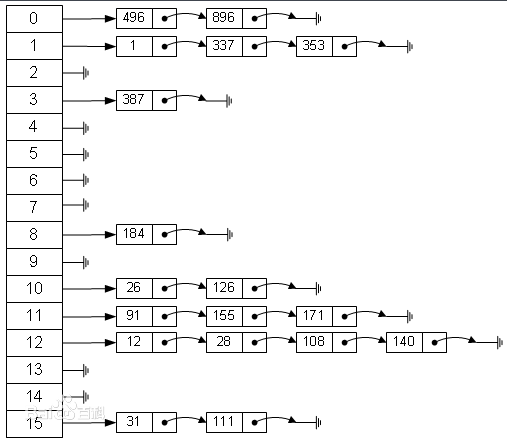2、开放地址法
如果遇到冲突的时候怎么办呢？就找hash表剩下空余的空间，找到空余的空间然后插入。就像你去商店买东西，发现东西卖光了，怎么办呢？找下一家有东西卖的商家买呗。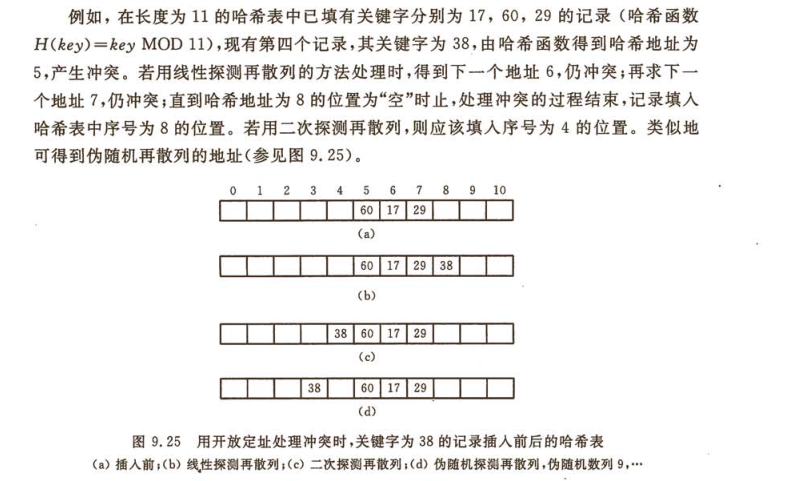## 2.哈希表的具体实现

2.1 链地址法实现的哈希表
（1）构建哈希表
首先定义链结点，以结构体Node展示，其中Node有三个属性，一个是key值，一个value值，还有一个是作为链表的指针，还有作为类的哈希表。

#define HASHSIZE 5

typedef struct Node{
char* key;
char* value;
Node *next;
}Node;

class HashTable{
private:
Node* node[HASHSIZE];
public:
HashTable();
~HashTable();
size_t hash(const char* key);
Node* lookup(const char* key);
bool install(const char* key,const char* value);
const char* get(const char* key);
void display();
private:
char* strdup(const char *str);     //给每个节点分配空间
};


（2）定义哈希表的构造方法、析构、节点空间分配算法

HashTable::HashTable(){
for (int i = 0; i < HASHSIZE; ++i)
{
node[i] = NULL;
}
}

HashTable::~HashTable(){
for (int i = 0; i < HASHSIZE; ++i)
{
Node *next = node[i];
while(next)
{
Node *tmp = next->next;
free(next->key);
free(next->value);
free(next);
next = tmp;
}
}
}

char* HashTable::strdup(const char *str){
int len=strlen(str)+1;
char *ns=(char*)malloc(len*sizeof(char));
strcpy(ns,str);
if(ns==nullptr)
return nullptr;
else
return ns;
}


（3）定义哈希表的Hash算法，在这里我使用time33算法

size_t HashTable::hash(const char* key){
size_t hash=0;
while (*key) {
hash += (hash << 5) + (*key++);
}
return hash%HASHSIZE;
}


（4）定义一个查找根据key查找结点的方法
思路：首先是用Hash函数计算头地址，然后根据头地址向下一个个去查找结点，如果结点的key和查找的key值相同，则匹配成功。

Node* HashTable::lookup(const char* key){
Node *np;
size_t index;
index = hash(key);
for(np=node[index];np;np=np->next){
if(!strcmp(key,np->key))
return np;
}
return NULL;
}


（5）定义一个插入结点的方法
思路：首先是查看该key值的结点是否存在，如果存在则更改value值就好，如果不存在，则插入新结点。

bool HashTable::install(const char* key,const char* value){
size_t index;
Node *np;
if(!(np=lookup(key))){
index = hash(key);
np = (Node*)malloc(sizeof(Node));
if(!np) return false;
np->key=strdup(key);
if(np->key == nullptr) return false;
np->next = node[index];       //设置新节点的下一个节点
node[index] = np;         //把新节点设置为头结点
}
else
{
free(np->value);
}
np->value=strdup(value);
if(np->key == nullptr) return false;
return true;
}


（6）打印出哈希表，用于Debug

void HashTable::display(){
Node* temp;
for (int i = 0; i < HASHSIZE; ++i)
{
if(!node[i]){
printf("[]\n");
}else{
printf("[");
for (temp=node[i]; temp; temp=temp->next)
{
printf("[%s][%s] ",temp->key,temp->value );
}
printf("]\n");
}
}
}


(7)测试代码是否正确

#include <stdio.h>
#include <stdlib.h>
#include <string.h>

void testHash(HashTable *ht)
{
const char* key[]={"a","b","c","d","e","f"};
const char* value[]={"value1","value2","value3","value4","value5","value6"};
for (int i = 0; i < 6; ++i)
{
ht->install(key[i],value[i]);
}
}
int main(int argc, char const *argv[])
{
HashTable ht;
testHash(&ht);
ht.display();
return 0;
}


输出如下:

[[d][value4] ]
[[e][value5] ]
[[f][value6] [a][value1] ]
[[b][value2] ]
[[c][value3] ]

展开全文数据结构和算法
• 哈希表的实现

# 一、哈希表

## 1.1 哈希概念

顺序结构以及平衡树中，元素关键码与其存储位置之间没有对应的关系，因此在查找一个元素时，必须要经过关键码的多次比较。顺序查找时间复杂度为O(N)，平衡树中为树的高度，即O( logN)，搜索的效率取决于搜索过程中元素的比较次数。
理想的搜索方法：可以不经过任何比较，一次直接从表中得到要搜索的元素。 如果构造一种存储结构，通过某种函数(hashFunc)使元素的存储位置与它的关键码之间能够建立一一映射的关系，那么在查找时通过该函数可以很快找到该元素。

当向该结构中：

• 插入元素
根据待插入元素的关键码，以此函数计算出该元素的存储位置并按此位置进行存放
• 搜索元素
对元素的关键码进行同样的计算，把求得的函数值当做元素的存储位置，在结构中按此位置取元素比较，若关键码相等，则搜索成功

该方式即为哈希(散列)方法，哈希方法中使用的转换函数称为哈希(散列)函数，构造出来的结构称为哈希表(Hash Table)(或者称散列表)

## 1.2 哈希冲突

对于两个数据元素的关键字ki 和 kj (i != j)，有ki != kj，但有：Hash(ki) == Hash(kj)，即：不同关键字通过相同哈希哈数计算出相同的哈希地址，该种现象称为哈希冲突或哈希碰撞。
把具有不同关键码而具有相同哈希地址的数据元素称为“同义词”。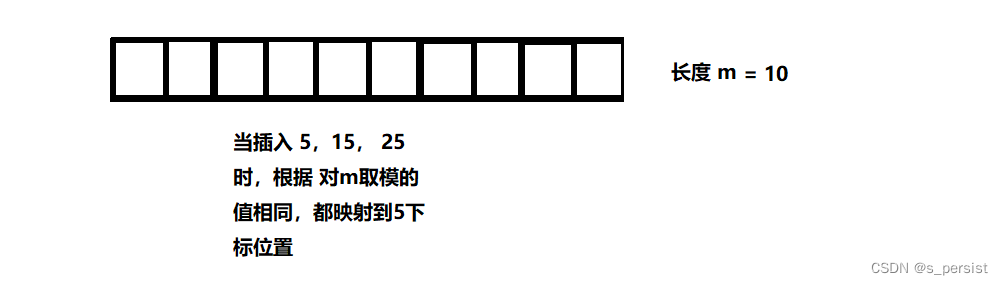## 1.3 哈希函数

引起哈希冲突的一个原因可能是：哈希函数设计不够合理。 哈希函数设计原则：
哈希函数的定义域必须包括需要存储的全部关键码，而如果散列表允许有m个地址时，其值域必须在0
到m-1之间.

哈希函数计算出来的地址能均匀分布在整个空间中,哈希函数应该比较简单。
常见哈希函数

1. 直接定制法–(常用)
取关键字的某个线性函数为散列地址：Hash（Key）= A*Key + B
优点：简单、均匀
缺点：需要事先知道关键字的分布情况 使用场景：适合查找比较小且连续的情况。
2. 除留余数法–(常用)
设散列表中允许的地址数为m，取一个不大于m，但最接近或者等于m的质数p作为除数，按照哈希函数：Hash(key) = key% p(p<=m),将关键码转换成哈希地址。
3. 平方取中法–(了解)
假设关键字为1234，对它平方就是1522756，抽取中间的3位227作为哈希地址； 再比如关键字为4321，对它平方就是18671041，抽取中间的3位671(或710)作为哈希地址 平方取中法比较适合：不知道关键字的分布，而位数又不是很大的情况。
4. 折叠法–(了解)
折叠法是将关键字从左到右分割成位数相等的几部分(最后一部分位数可以短些)，然后将这几部分叠加求和，并按散列表表长，取后几位作为散列地址。
折叠法适合事先不需要知道关键字的分布，适合关键字位数比较多的情况。
5. 随机数法–(了解)
选择一个随机函数，取关键字的随机函数值为它的哈希地址，即H(key) = random(key),其中random为随机数函数。通常应用于关键字长度不等时采用此法。
6. 数学分析法–(了解)
设有n个d位数，每一位可能有r种不同的符号，这r种不同的符号在各位上出现的频率不一定相同，可能在某些位上分布比较均匀，每种符号出现的机会均等，在某些位上分布不均匀只有某几种符号经常出现。可根据散列表的大小，选择其中各种符号分布均匀的若干位作为散列地址。

哈希函数设计的越精妙，产生哈希冲突的可能性就越低，但是无法避免哈希冲突

## 1.4 载荷因子

散列表的载荷因子定义为：a = 填入表中的元素个数 / 散列表的长度

a 是散列表装满程度的标志因子。由于表长是定值，a 与“填入表中的元素个数”成正比，所以 a 越大，表明填入表中的元素越多，产生冲突的可能性就越大；反之，a 越小，标明填入表中的元素越少，产生冲突的可能性就越小。实际上，散列表的平均查找长度是载荷因子 a 的函数，只是不同处理冲突的方法有不同的函数。

对于开放定址法，荷载因子是特别重要因素，应严格限制在0.7-0.8以下。超过0.8，查表时的CPU缓存不命中（cache missing）按照指数曲线上升。因此，一些采用开放定址法的hash库，如Java的系统库限制了荷载因子为0.75，超过此值将resize散列表。

# 二、解决哈希冲突

解决哈希冲突两种常见的方法是：闭散列和开散列

## 2.1 闭散列

闭散列：也叫开放定址法，当发生哈希冲突时，如果哈希表未被装满，说明在哈希表中必然还有空位置，那么可以把key存放到冲突位置中的“下一个” 空位置中去。那如何寻找下一个空位置呢？

1. 线性探测
从发生冲突的位置开始，依次向后探测，直到寻找到下一个空位置为止。
比如上面的冲突，首先5放在5下标位置，15映射后发生冲突，从5下标往后面找，找到一个空位置就可以放了。
• 插入
通过哈希函数获取待插入元素在哈希表中的位置，如果该位置中没有元素则直接插入新元素，如果该位置中有元素发生哈希冲突，使用线性探测找到下一个空位置，插入新元素
• 删除
采用闭散列处理哈希冲突时，不能随便物理删除哈希表中已有的元素，若直接删除元素会影响其他元素的搜索。比如删除元素4，如果直接删除掉，44查找起来可能会受影响。因此线性探测采用标记的伪删除法来删除一个元素。

线性探测优点：实现非常简单
线性探测缺点：一旦发生哈希冲突，所有的冲突连在一起，容易产生数据“堆积”，即：不同关键码占据了可利用的空位置，使得寻找某关键码的位置需要许多次比较，导致搜索效率降低。

1. 二次探测
线性探测的缺陷是产生冲突的数据堆积在一块，这与其找下一个空位置有关系，因为找空位置的方式就是挨着往后逐个去找，因此二次探测为了避免该问题，找下一个空位置的方法为：Hi= (H0 + i2)% m，或者：Hi = (H0 - i2)% m。其中：i = 1,2,3…， H0是通过散列函数Hash(x)对元素的关键码 key 进行计算得到的位置，m是表的大小。

## 2.2 闭散列代码实现

• 删除某一个数据会影响其他很多的（与之相关发生冲突的数据）数据，所以使用标记法，表示已被删除，这里使用枚举表示
enum Status
{
EXIST,
EMPTY,
DELETE
};

• 定义数据结点，这里存储的是键值对

template<class K, class V>
struct HashData
{
pair<K, V> _kv;
Status _status = EMPTY;
};

• 由于传入的key值可能不是自然数，比如常用的字符串类型，它是不能取模，需要使用字符串哈希，变成一个整数来取模。
所以需要设计一个仿函数拿到对应的数值
// 哈希表数据结构
template<class K, class V, class HashFunc = Hash<K> >
class HashTable
{
private:
vector<HashData<K, V> > _tables;
size_t _n = 0; // 哈希表中存放的个数
}

// 用于将整数转换成0到大于零的正整数，防止出现对负数取模
template<class K>
struct Hash
{
// 将整型转成正数
size_t operator()(const K& key)
{
return key;
}
};
// 常用到字符串类型的key值，这是一个特化版本
template<>
struct Hash <string>
{
// 字符串哈希
size_t operator()(const string& s)
{
size_t value = 0;
for (auto ch : s)
{
// 也可以乘以31、131、1313、13131、131313..
// 乘上一个数，减少冲突的概率
value *= 31;
value += ch;
}
return value;
}
};

• 扩容
当负载因子较大时要扩容，防止出现冲突聚集，效率下降
// 扩容，负载因子大于7时
if (_tables.size() == 0 || _n * 10 / _tables.size() >= 7)
{
size_t newSize = _tables.size() == 0 ? 10 : _tables.size() * 2;
// 扩容有两种方法
// 1. 可以遍历一遍数组，并重新映射
// 2. 创建一个本类对象，调用它的插入函数，完成映射，再交换对象
HashTable<K, V, HashFunc> newHT;
newHT._tables.resize(newSize);
for (int i = 0; i < _tables.size(); i++)
{
if (_tables[i]._status == EXIST)
{
newHT.Insert(_tables[i]._kv);
}
}
// 交换两个数组
_tables.swap(newHT._tables);
}

• 完整实现
namespace CloseHash
{
enum Status
{
EXIST,
EMPTY,
DELETE
};
template<class K, class V>
struct HashData
{
pair<K, V> _kv;
Status _status = EMPTY;
};
// 用于将整数转换成0到大于零的正整数，防止出现对负数取模
template<class K>
struct Hash
{
size_t operator()(const K& key)
{
return key;
}
};
// 常用到字符串类型的key值，这是一个特化版本
template<>
struct Hash <string>
{
// 字符串哈希
size_t operator()(const string& s)
{
size_t value = 0;
for (auto ch : s)
{
// 也可以乘以31、131、1313、13131、131313..
// 乘上一个数，减少冲突的概率
value *= 31;
value += ch;
}
return value;
}
};

template<class K, class V, class HashFunc = Hash<K> >
class HashTable
{
private:
vector<HashData<K, V> > _tables;
size_t _n = 0;
public:
bool Erase(const K& key)
{
HashData<K, V>* ret = Find(key);
if (ret == nullptr)
return false;
else
{
--_n;
ret->_status = DELETE;
return true;
}
}
HashData<K, V>* Find(const K& key)
{
if (_tables.size() == 0)
{
return nullptr;
}
HashFunc hf;
// 线性探测 or 二次探查
int i = 0;
int start = hf(key) % _tables.size();
int index = start;
while (_tables[index]._status != EMPTY)
{
if (_tables[index]._status == EXIST && _tables[index]._kv.first == key)
return &_tables[index];
index++; // 线性探测
//index = start + i * i ++ ;// 二次探查
index %= _tables.size();
}

return nullptr;

}
bool Insert(const pair<K, V>& kv)
{
HashData<K, V>* ret = Find(kv.first);
if (ret != nullptr)
{
return false;
}

// 扩容，负载因子大于7时
if (_tables.size() == 0 || _n * 10 / _tables.size() >= 7)
{
size_t newSize = _tables.size() == 0 ? 10 : _tables.size() * 2;
// 扩容有两种方法
// 1. 可以遍历一遍数组，并重新映射
// 2. 创建一个本类对象，调用它的插入函数，完成映射，再交换对象
HashTable<K, V, HashFunc> newHT;
newHT._tables.resize(newSize);
for (int i = 0; i < _tables.size(); i++)
{
if (_tables[i]._status == EXIST)
{
newHT.Insert(_tables[i]._kv);
}
}
// 交换两个数组
_tables.swap(newHT._tables);
}

HashFunc hf;
// 线性探测 or 二次探查
int i = 0;
int start = hf(kv.first) % _tables.size();
int index = start;
while (_tables[index]._status == EXIST)
{
index++; // 线性探测
//index = start + i * i ++ ;// 二次探查
index %= _tables.size();
}
_tables[index]._kv = kv;
_tables[index]._status = EXIST;
++_n;

return true;
}
};
}


## 2.3 开散列

开散列

1. 开散列概念
开散列法又叫链地址法(拉链法)，首先对关键码集合用散列函数计算散列地址，具有相同地址的关键码归于同一子集合，每一个子集合称为一个桶，各个桶中的元素通过一个单链表链接起来，各链表的头结点存储在哈希表中。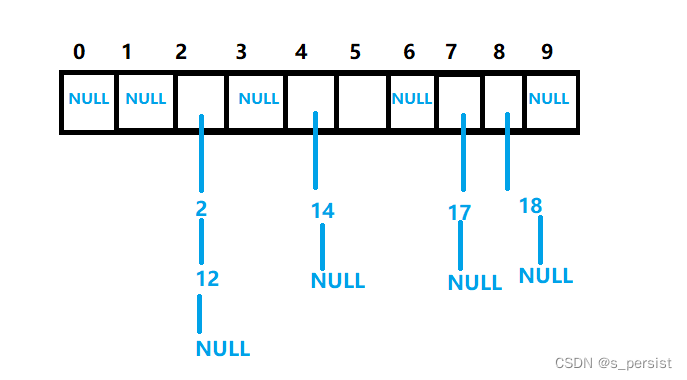开散列中每个桶中放的都是发生哈希冲突的元素。

## 2.4 开散列代码实现

• 开散列数据结构
可以是一个数组下面挂一个单链表
// 单链表结点数据
template<class K, class V>
struct HashData
{
pair<K, V> _kv;
HashData<K, V>* _next;

HashData(const pair<K, V>& kv)
:_kv(kv), _next(nullptr)
{}
};

template<class K, class V, class HashFunc = Hash<K> >
class HashTable
{
typedef HashData<K, V> Node;
private:
vector<Node*> _tables;
size_t _n = 0; // 有效数字个数
}

• 扩容
// 载荷因子为1时扩容
if (_tables.size() == 0 || _n * 10 / _tables.size() == 10)
{
// 因为哈希表每个元素下挂一个单链表，它们的空间是动态申请的，只有指针管理
// 所以使用上面的方法（创建一个新大小的类，调用插入函数，最后交换）没有直接交换结点快，不用申请空间
size_t newSize = _tables.size() == 0 ? 10 : _tables.size() * 2;
vector<Node*> newTables(newSize);
for (size_t i = 0; i < _tables.size(); i++)
{
Node* cur = _tables[i];
while (cur)
{
Node* prev = cur;
// 链接到新的表上
size_t newIndex = hf(cur->_kv.first) % newSize;
cur->_next = newTables[newIndex];
newTables[newIndex] = cur;
cur = prev->_next;
}
_tables[i] = nullptr;
}
_tables.swap(newTables);
}

• 完整代码
namespace LinkHash
{
template<class K, class V>
struct HashData
{
pair<K, V> _kv;
HashData<K, V>* _next;

HashData(const pair<K,V>& kv)
:_kv(kv), _next(nullptr)
{}
};
// 用于将整数转换成0到大于零的正整数，防止出现对负数取模
template<class K>
struct Hash
{
// 将整型转成正数
size_t operator()(const K& key)
{
return key;
}
};
// 常用到字符串类型的key值，这是一个特化版本
template<>
struct Hash <string>
{
// 字符串哈希
size_t operator()(const string& s)
{
size_t value = 0;
for (auto ch : s)
{
// 也可以乘以31、131、1313、13131、131313..
// 乘上一个数，减少冲突的概率
value *= 31;
value += ch;
}
return value;
}
};
template<class K, class V, class HashFunc = Hash<K> >
class HashTable
{
typedef HashData<K, V> Node;
private:
vector<Node*> _tables;
size_t _n = 0; // 有效数字个数
public:
bool Erase(const K& key)
{
Node* res = Find(key);
if (res == nullptr) return false;

HashFunc hf;
//用于单链表的删除，存储前一个结点的地址
int index = hf(key) % _tables.size();
Node* cur = _tables[index], *prev = nullptr;
while (cur)
{
if (cur->_kv.first == key)
{
if (prev == nullptr) // 头删
{
_tables[index] = cur->_next;
}
else // 中间删
{
prev->_next = cur->_next;

}
delete cur;

--_n;
return true;
}
else
{
prev = cur;
cur = cur->_next;
}
}
return false;
}
Node* Find(const K& key)
{
if (_n == 0)
return nullptr;
HashFunc hf;
int index = hf(key) % _tables.size();
Node* cur = _tables[index];
while (cur)
{
if (cur->_kv.first == key) return cur;
else cur = cur->_next;
}
return nullptr;
}
bool Insert(const pair<K, V>& kv)
{
Node* res = Find(kv.first);
if (res != nullptr) return false;
HashFunc hf;

// 载荷因子为1时扩容
if (_tables.size() == 0 || _n * 10 / _tables.size() == 10)
{
// 因为哈希表每个元素下挂一个单链表，它们的空间是动态申请的，只有指针管理
// 所以使用上面的方法（创建一个新大小的类，调用插入函数，最后交换）没有直接交换结点快，不用申请空间
size_t newSize = _tables.size() == 0 ? 10 : _tables.size() * 2;
vector<Node*> newTables(newSize);
for (size_t i = 0; i < _tables.size(); i++)
{
Node* cur = _tables[i];
while (cur)
{
Node* prev = cur;
// 链接到新的表上
size_t newIndex = hf(cur->_kv.first) % newSize;
cur->_next = newTables[newIndex];
newTables[newIndex] = cur;
cur = prev->_next;
}
_tables[i] = nullptr;
}
_tables.swap(newTables);
}

int index = hf(kv.first) % _tables.size();
// 头插法
Node* newNode = new Node(kv);
newNode->_next = _tables[index];
_tables[index] = newNode;
++_n;
}
};
void TestHashTable()
{
int a[] = { 4, 24, 14,7,37,27,57,67,34,14,54 };
HashTable<int, int> ht;
for (auto e : a)
{
ht.Insert(make_pair(e, e));
}

ht.Erase(24);
ht.Erase(67);
ht.Insert(make_pair(84, 84));
}
}


展开全文散列表
• ## 哈希链表的实现C++

千次阅读 2021-12-03 13:25:05
哈希链表的实现

两大类，一类是结点的定义，一类是哈希表的定义：具体的算法实现可以参考下面的程序：

.hpp文件

#pragma once
#include<iostream>
#include<ctype.h>

using namespace std;

typedef int Elemtype;
const int DEFAULT_SIZE=5;//如果没有指定桶的个数，就按默认值5

class Lnode
{
public:
bool IsEmpty()
{
if (this->next== nullptr)
return true;
else
return false;
}
public:
Lnode* next;
int key;
Elemtype data;
};

class Hash_Table
{

public:
Hash_Table();
int Hash_fun(Elemtype value);//定义哈希函数
void Create_HashTable(Elemtype* value, int size);//创建哈希表
bool unique_Lnode(Elemtype value); //判断结点是否重复
void Insert_Lnode(Elemtype value);//插入结点进入哈希表
void Debug();//测试一下
~Hash_Table();
private:
Lnode*table;
int num;
};

Hash_Table::Hash_Table()
{
this->num = DEFAULT_SIZE;
cout << "输入哈希桶的个数："; cin >>this-> num;
if (num <= 0)
this->num = DEFAULT_SIZE;
table = new Lnode[num];
for (int i = 0; i < this->num; i++)
{
this->table[i].data = NULL;
this->table[i].key=i;
this->table[i].next = nullptr;
}
}

int Hash_Table::Hash_fun(Elemtype value)
{
return (value % this->num);
}
void Hash_Table::Create_HashTable(Elemtype* value,int size)
{
for (int i = 0; i < size; i++)
{
int _key = this->Hash_fun(value[i]);
if (table[_key].IsEmpty())
{
Lnode* node = new Lnode;
node->data = value[i]; node->key = _key; node->next = nullptr; table[_key].next = node;
}
else//如果现在结点不为空，先去遍历，看是否该元素已经添加
{
bool reslut= this->unique_Lnode(value[i]);//reslut为1：该元素为新元素，为0，就不再添加了
if (reslut)
{
Lnode* node = new Lnode;
node->data = value[i]; node->key = _key; node->next = table[_key].next; table[_key].next = node;
}
else
continue;
}
}
}

bool Hash_Table::unique_Lnode(Elemtype value)//判断数据是否已经存在
{
int index = this->Hash_fun(value);
Lnode *temp = table[index].next;
while (temp)
{
if (temp->data == value)
return false;
temp = temp->next;
}
return true;
}

void Hash_Table::Insert_Lnode(Elemtype value)
{
int reslut = this->Hash_fun(value);
if (this->table[reslut].next==nullptr)
{
Lnode* node = new Lnode;
node->data = value; node->key = reslut; node->next = nullptr; table[reslut].next = node;
}
else//如果现在结点不为空，先去遍历，看是否该元素已经添加
{
bool reslut1 = this->unique_Lnode(value);//reslut为1：该元素为新元素，为0，就不再添加了
if (reslut1)
{
Lnode* node = new Lnode;
node->data = value; node->key = reslut; node->next = table[reslut].next; table[reslut].next = node;//前插法
}
else
return;
}
}

void Hash_Table::Debug()
{
cout << "测试如下：" << endl;
for (int j = 0; j < this->num; j++)
{
Lnode *temp = this->table[j].next;
cout << "索引为" << j << "的数值：";
if (temp == nullptr)
{
cout << "NULL" << endl;
continue;
}
while (temp)
{
cout << temp->data << "\t";
temp = temp->next;
if (!temp)
cout << endl;
}
}
}

Hash_Table::~Hash_Table()//尤其要注意开辟在堆区的数据的删除
{
for (int j = 0; j < this->num; j++)
{
Lnode* p1,*p2;
p1 = this->table[j].next;
p2 = p1;
while (p1)
{
p2 = p1->next;
delete p1;
p1 = p2;
}
this->table[j].next = nullptr;
}
delete[] this->table;
cout << "释放空间成功！" << endl;
}

main函数：

#include<iostream>
#include"哈希表.hpp"
using namespace std;

void text()
{
Elemtype ch[] = { 54,596,45,12,56,47,12,59,45,33,33,21,45,96,14,96,77,54,44,66,23,44 };
int length = sizeof(ch) / sizeof(ch);
Hash_Table H1;
H1.Create_HashTable(ch, length);
H1.Debug();
cout << "--------------------------------------" << endl;
H1.Insert_Lnode(888);
H1.Debug();
cout << "--------------------------------------" << endl;
}

int main()
{
text();
system("pause");
return 0;
}

测试的结果：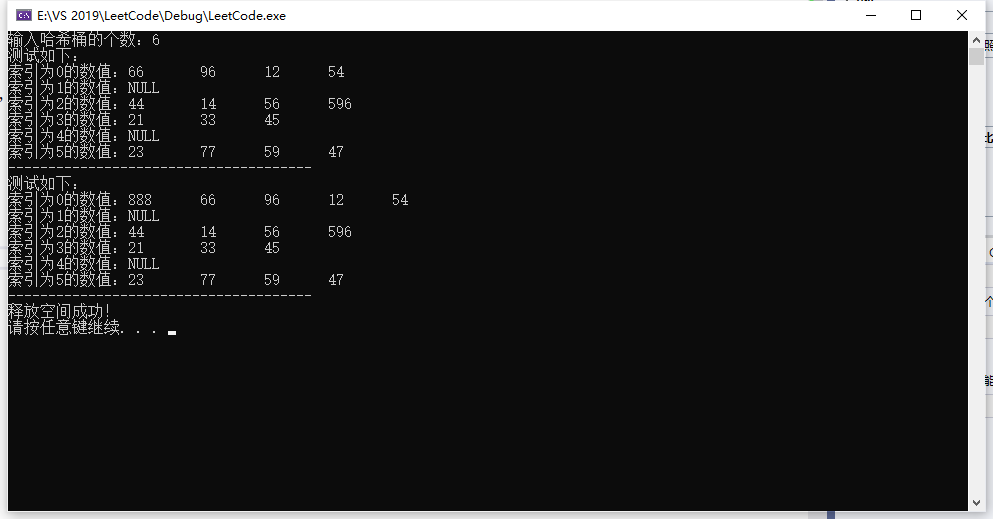### 讨论：

这里主要是针对int型建立的哈希表，哈希函数相对比较简单，对字符串等其他类型，需要改动程序。

展开全文链表
• ## C++模拟实现哈希表

多人点赞 2022-05-22 19:22:20散列表 数据结构 c语言
• ## C++哈希表

千次阅读 2022-03-25 22:42:59
哈希表 哈希表(hash table)：根据关键字(Key)而直接进行访问值(Value)的数据结构。 一般我们可以用哈希表来快速判断一个元素是否出现在集合里。 以数组为例，数组也是哈希表——哈希表关键码即数组的索引，这样...
• ## 哈希表、哈希桶（C++实现）

千次阅读 多人点赞 2022-01-23 19:41:13
哈希表、哈希桶哈希概念哈希函数哈希冲突解决哈希冲突闭散列线性探测闭散列的实现哈希表的结构哈希表的插入哈希表的查找哈希表的删除开散列哈希概念 哈希概念 在顺序结构和平衡树中，元素关键码与其存储位置之间没有...散列表
• 模拟实现哈希表两万字详解，包括哈希表的闭散列实现和开散列实现，以及迭代器的实现。散列表 数据结构
• 在刷初级算法的字符串有好多关于哈希表的内容 所以作词笔记，强化哈希。 首先哈希表的作用是什么？它可以快速的查找。key-value值。 key值是唯一的。就例如一个统计某几人的身高。 如下是STL标准库map容器的实例。...散列表
• 如果现在做哈希表的题目，是因为按专题刷的哈希表的题目，所以会直接用哈希表。但是遇到一道新的题目，没有标签，怎么想到使用哈希表呢？在unordered_set跟unordered_map中删除元素，都用。如果是unordered_map，...散列表
• 哈希表哈希表概念链式存储实现顺序存储实现实际应用字串匹配hash_table.htest.cpp 哈希表 概念 哈希表-散列表， 它是基于快速存储的角度设计的，也是一种典型的“空间换时间”的做法。 (键值(编号)就代表了这个数据...数据结构
•算法 leetcode
•散列表 数据结构
• hash的简单实现 数据结构初学 对于这里希望留下一些资源 期待大家批评指正
•数据结构 算法 散列表
• 用字符串做key的哈希表，采用瘦模板技术，不会导致代码膨胀，可以自动扩展
• 哈希表c++实现，数据结构课程资源，课上独立完成，供大家借鉴数据结构
• 本章主要讲解了两种常见的哈希.学完本章,相信你会对一般的哈希算法有一定的了解。散列表 算法
• 这个代码是我用C++编写的队列实现，实现方式是数组的形式，对于刚学习数据结构的人来说里面的功能比较全面
• public成员包括构造函数、析构函数和复制构造函数以及=重载函数，其它成员函数主要有：traver(遍历散列表)、show()(打印出哈希表所存的元素)返回值为bool类型的函数search\insert\Delete。search函数（查询关键字为...
• C++实现哈希表创建、查找、显示、计算ALS（详细）。针对某个数值序列，设计一个哈希表，完成相应的建表和查表顺序。哈希函数用除留余数法构造，用线性探测再散列的方法处理哈希地址冲突。针对给定的数列：{23,5,17,...数据结构 算法
• ## 【C++】构建哈希表

千次阅读 2021-04-01 15:08:05
哈希表类型也可以更改，比如 key 和 value 都是 char 类型： unordered_map, char> map={ {')','('}, {']','['}, {'}','{'} }; 在调用哈希表时，注意使用方括号，而不是圆括号 cout ['A']; 如果需要判断某 key 是否...
• 哈希表 散列表,它是基于快速存取的角度设计的,也是一种典型的 “空间换时间” 的做法; 键(key) : 组员的编号, 如: 1,2,3…; 值(value): 组员的其他信息 (包含: 性别, 年龄, 战斗力等); 索引: 数组的下标 (0,1,2…), ...mysql c语言
• 使用map map是STL的一个关联容器，它提供一对一的hash。 第一个可以称为关键字(key)，每个关键字只能在...比如需要组合的比较数组中的两个数据时，用哈希表可以将遍历过得数据存下来节省遍历搜索的时间 例题如下...sql
• C++98中，STL提供了底层为红黑树结构的一系列关联式容器，在查询时效率可达到 ，...5.哈希概念 顺序结构以及平衡树中，元素关键码与其存储位置之间没有对应的关系，因此在查找一个元素时，必须要经过关键码的多次比较
• 主要介绍了C++中的哈希容器unordered_map使用示例,本文直接给出实例代码,并讲解了一些hash table的知识,需要的朋友可以参考下...

# 哈希表c++c++ 订阅